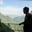Related Tags

r programming
communitycreator

# What are bar plots in R programming?AKASH BAJWA

A bar plot displays data in rectangular bars, with the lengths of the bars proportional to the value of the variable. R uses the barplot() subroutine to generate bar plots. R generates both types of bar plots, vertical and horizontal. You can pass different arguments to customize the barplot() function.

### Syntax

The prototype of the barplot() function is as follows:

barplot(h, xlab, ylab, main, names.arg, col)


### Parameters

The barplot() function accepts the following parameters:

• h: a matrix that contains numeric values of the chart
• xlab: a tag for the x-axis
• ylab: a tag for the y-axis
• main: the title for bar plot
• names.arg: a vector of labels that appear in each bar
• col: specifies the colors of the bars

### Examples

#### Bar plot without title or tags

The code below plots a simple bar plot. We pass the data vector as an argument to the barplot() method to generate a graph.

# Create the data for the chart
data <- c(8,13,26,3,43,21)

# Give the chart file a name
png(file = "barplot.png")

# Plot the bar chart
barplot(data)

#### Bar plot with title and tags

In this example, the bar plot contains x and y labels, as well as a graph name. The code below plots a graph that represents the population of different states in the United States.

# Create the data
data <- c(8,13,26,3,43,21)
labels <- c("Calif.", "Colo.", "Wash.", "Fla.", "Ga.", "Tex.")
# Plot
barplot(data, xlab= "States", ylab = "Population in 1000s", main="Population Chart",names.arg = labels, col ="red", border = "yellow")
# Give a name to file
png(file = "US_States.png")

Note: The png(file = "US_States.png") command saves the bar plot as a file named US_States.png.

### Types of bar plots in R

R programming offers multiple types of bar plots, such as:

1. Simple colored bar plot
2. Horizontal bar plot
3. Bar plot with labels
4. Stacked bar plot with legend
5. Grouped bar plot with legend

#### 1. Simple colored bar plot

The code below plots a simple bar plot. We pass the data vector as an argument to the barplot() method to generate a blue graph.

# Create data
data <- c(0.2, 0.75, 0.2, 0.4, 0.5)
barplot(data, col = "#3375FF")
# Give a name to file
png(file = "graph.png")

#### 2. Horizontal bar plot

To plot the graph horizontally, the horiz parameter must be set to TRUE, as shown below:

# Horizontal barplots
data <- c(0.2, 0.65, 0.3, 0.4, 0.5)
barplot(data, horiz = TRUE)
# Give a name to file
png(file = "graph.png")

#### 3. Bar plot with labels

Each bar can also be given a specific name or label by setting the names.arg attribute equal to the desired bar labels, e.g., labels <- LETTERS[1:6].

The LETTERS[1:6] command generates English letters from A to F, as shown below:

# Create grouping variable
data <- c(0.2, 0.65, 0.3, 0.4, 0.5,0.8)
labels <- LETTERS[1:6]
barplot(data, names.arg =labels)
# Give a name to file
png(file = "graph.png")

#### 4. Stacked bar plot with a legend

To generate a stacked bar plot with a legend, you can use the legend() method with specific parameters, as shown below:

# Create matrix for stacked barplot
data <- as.matrix(data.frame(A = c(0.9, 1.2), B = c(0.6, 0.3), C = c(0.6, 0.3), D = c(0.4, 0.4), E = c(0.3, 0.1), F = c(0.3, 0.1)))
# Create stacked barplot
barplot(data, col = c("#3375FF", "#494B4F"))
legend("topright", legend = c("Covid Patients", "Pneumonia Patients"), fill = c("#3375FF", "#494B4F"))
# Give a name to file
png(file = "graph.png")

#### 5. Grouped bar plot with a legend

Grouped bar plots can be used to compare multiple instances together. The code below compares $5$ rounds of soccer matches between Bolivia and Paraguay.

data <- as.matrix(data.frame(R1 = c(0.9, 1.2), R2 = c(0.6, 0.3), R3 = c(0.6, 0.3), R4 = c(0.4, 0.4), R5 = c(0.3, 0.1)))
# Create grouped barplot
barplot(data,  col = c("#FF3333", "#3375FF"), beside = TRUE)
legend("topright", legend = c("Bolivia", "Paraguay"), fill = c("#FF3333", "#3375FF"))
# Give a name to file
png(file = "graph.png")

RELATED TAGS

r programming
communitycreator

CONTRIBUTORAKASH BAJWA
RELATED COURSES

View all Courses

Keep Exploring

Learn in-demand tech skills in half the time